Test Paper includes questions from Counting of Figure and Missing Number topic of Reasoning. This test is useful for SSC, RRB and other Govt Jobs.

Reasoning – COUNTING OF FIGURE AND MISSING NUMBER

Number of Question : 27 Time 25 Minute

Click on Start Button to start the Mock Test, useful for Online Exam Practice.

8
Created on

Reasoning Mock Test – Counting of Figure and Missing Number

Reasoning Mock Test on Counting of Figure and Missing Number

1 / 27

Count Number of Straight lines

`सीधी रेखाओं की संख्या गिनें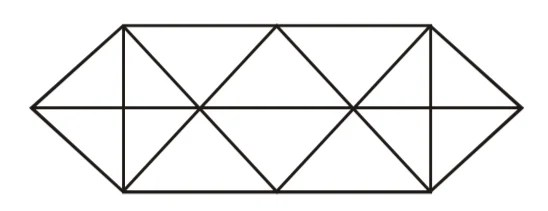`

2 / 27

Count the number of Cube3 / 27

Count the number of Circle

वृत्त की संख्या गिनें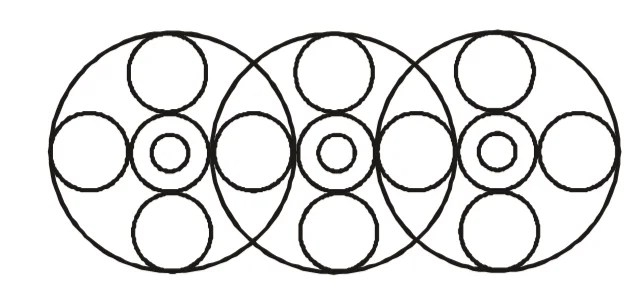4 / 27

Counting of number of Rectangles

`आयतों की संख्या गिनें`5 / 27

Count Number of Rectangles

`आयतों की संख्या गिनें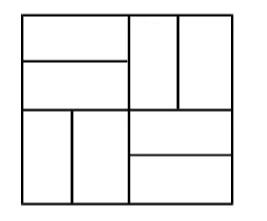`

6 / 27

Count the number of rectangles

`आयतों की संख्या गिनें`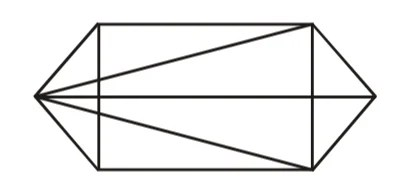7 / 27

Count the number of squares

`वर्गों की संख्या की गणना करें`8 / 27

Count the Triangles in the given figure

त्रिभुजों को गिनें9 / 27

Count the triangle in the figure.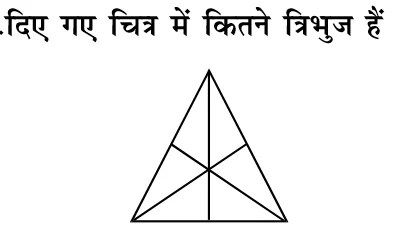10 / 27

Count the number of triangle in the given figure.11 / 27

counting of Rectangle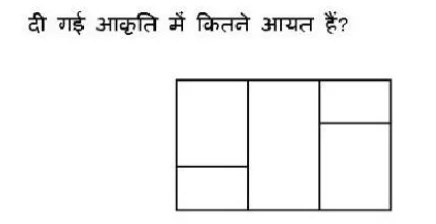12 / 27

Count the number of triangles in the figure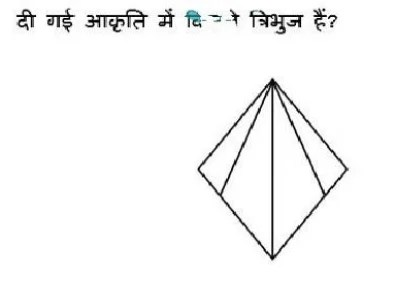13 / 27

Count the number of Triangle in the figure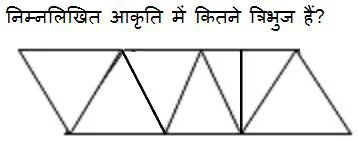14 / 27

Count the Triangles15 / 27

Count the rectangles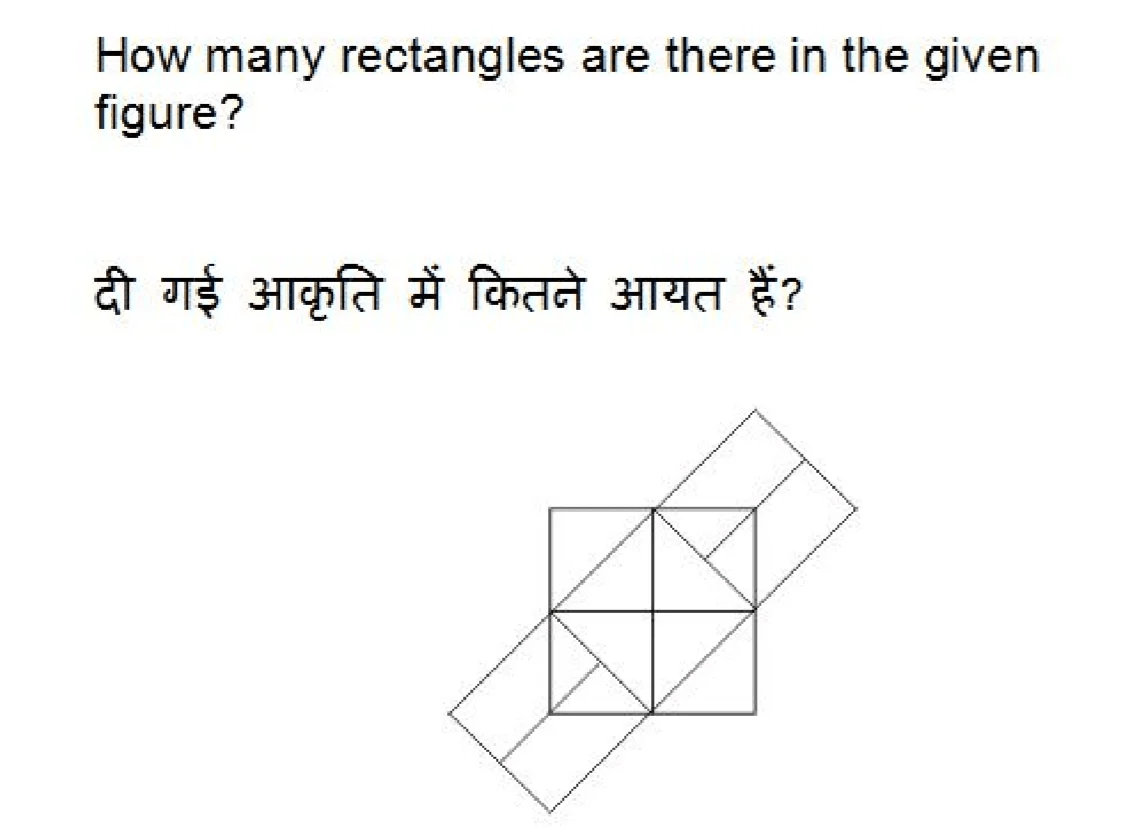16 / 2717 / 27

`Find the Missing Number,  गुम संख्या का पता लगाएं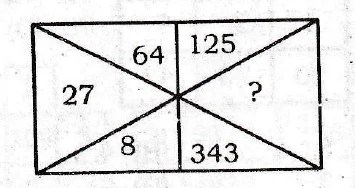`

18 / 27

`Find Missing Number गुम संख्या का पता लगाएं`

19 / 27

Find Missing Number

`गुम संख्या का पता लगाएं`

20 / 27

`Find Missing Numberगुम संख्या का पता लगाएं`

21 / 27

Find the Missing number,

`गुम संख्या का पता लगाएं`22 / 27

Find the Missing number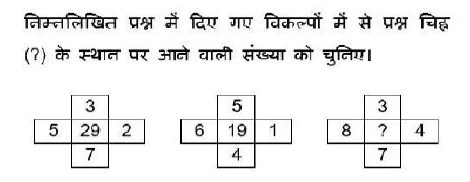23 / 27

Find Missing number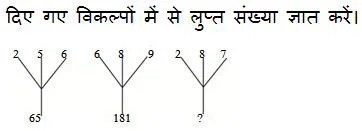24 / 27

Find Missing number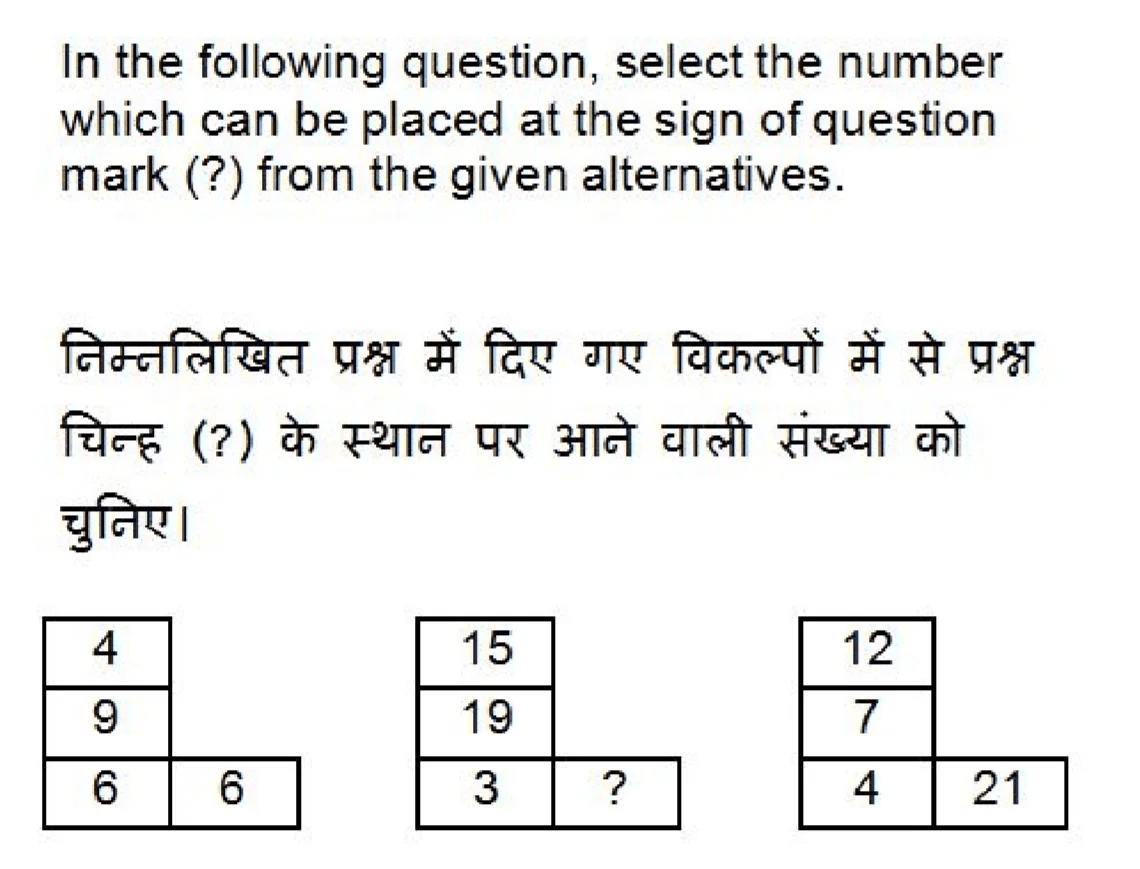25 / 27

Find Missing Number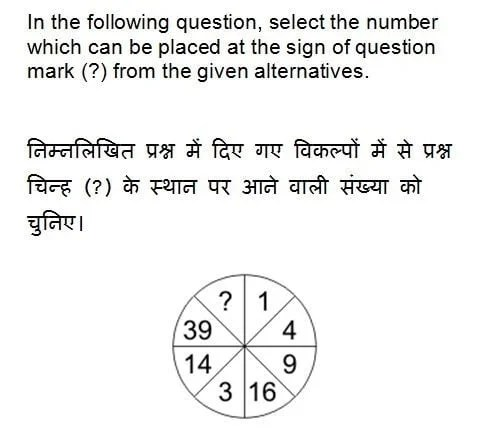26 / 2727 / 27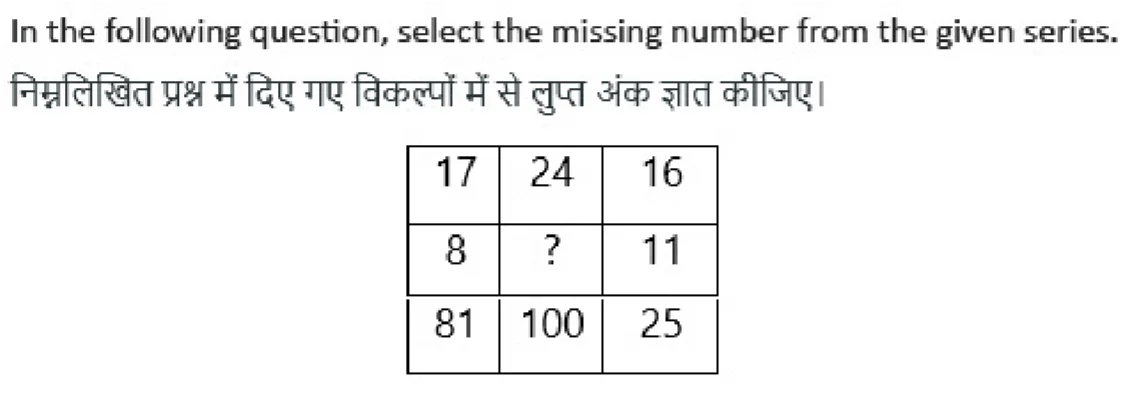0%

### COUNTING OF FIGURE AND MISSING NUMBER : Reasoning Test

The above Practice test includes question about the counting of following figures

• Count Number of Straight lines
• सीधी रेखाओं की संख्या गिनें
• Count the number of Cube
• घन की संख्या गिनें
• Count the number of Circle
• वृत्त की संख्या गिनें
• Counting of number of Rectangles
• आयतों की संख्या गिनें
• Count the number of squares
• वर्गों की संख्या की गणना करें
• Count the Triangles in the given figure
• त्रिभुजों को गिनें
• Find the Missing Number,
• गुम संख्या का पता लगाएं

### Reasoning Mock Test : Topic Wise

Super Success Muzaffarnagar SSC Study Location Map Bypass Flyover, Jansath Road offer following Classes and courses:

• Coaching Classes for Govt Jobs and
• Tuition CBSE and UP Board – NCERT English and Hindi Medium
• Home tutorial in Muzaffarnagar
• Further, Conduct CCC, Basic and ‘O’ Level computer Course

Moreover, Super Success approved by NIELIT (DOEACC) for conducting computer Courses in Muzaffarnagar